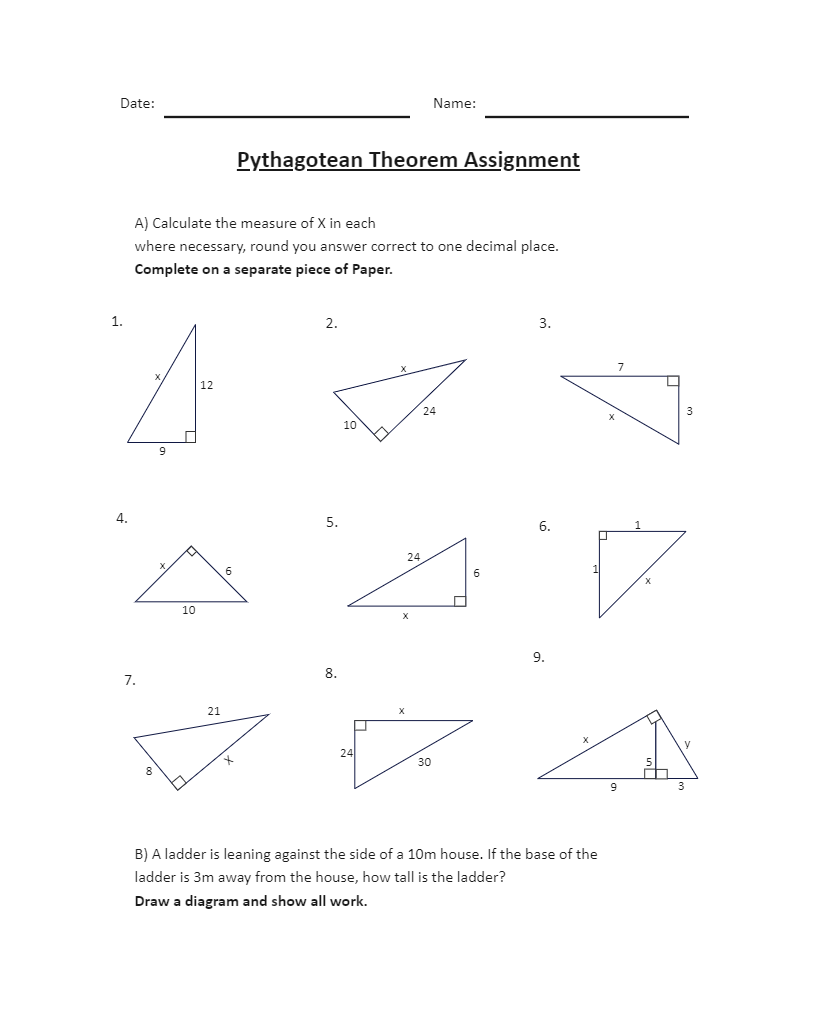Template Community / Pythagorean Theorem Worksheet Example

# Pythagorean Theorem Worksheet Example

Share
Duplicate0
0
Report
Publish time：02-09-2022
As most of us know, the Pythagorean theorem is a fundamental relation in geometry among the three sides of a right triangle. Teaching your students about the Pythagorean Theorem will help them strengthen their algebra and geometry as they study further. The Pythagorean Theorem states that the area of the square whose side is the hypotenuse equals the sum of the areas of the squares on the other two sides. Following is the Pythagorean Theorem Assignment, where the students have to calculate the measure of X in each diagram. In addition to this, there is a practical question also being put out for the students to solve and answer.
CreatorKiraaaaPost
Recommended TemplatesPublish
Your
Diagram